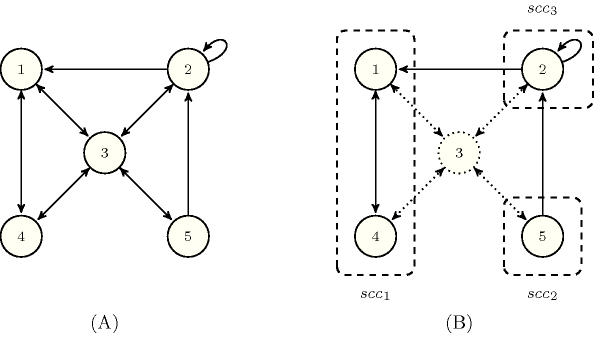### 3.7.246. Strong articulation point

A constraint for which the filtering algorithm uses the notion of strong articulation point. A strong articulation point of a strongly connected digraph $G$ is a vertex such that if we remove it, $G$ is broken into at least two strongly connected components. Figure 3.7.75 illustrates the notion of strong articulation point on the digraph depicted by part (A). The vertex labelled by 3 is a strong articulation point since its removal creates the three strongly connected components depicted by part (B) (i.e., the first, second and third strongly connected components correspond respectively to the sets of vertices $\left\{1,4\right\}$, $\left\{2\right\}$ and $\left\{5\right\}$). From an algorithmic point of view, it was shown in [ItalianoLauraSantaroni10] how to compute all the strong articulation points of a digraph $G$ in linear time with respect to the number of arcs of $G$.

##### Figure 3.7.75. (A) A connected digraph, (B) its three strongly connected components ${\mathrm{𝑠𝑐𝑐}}_{1}$, ${\mathrm{𝑠𝑐𝑐}}_{2}$ and ${\mathrm{𝑠𝑐𝑐}}_{3}$ when its unique strong articulation point (i.e., the vertex labelled by 3) is removed